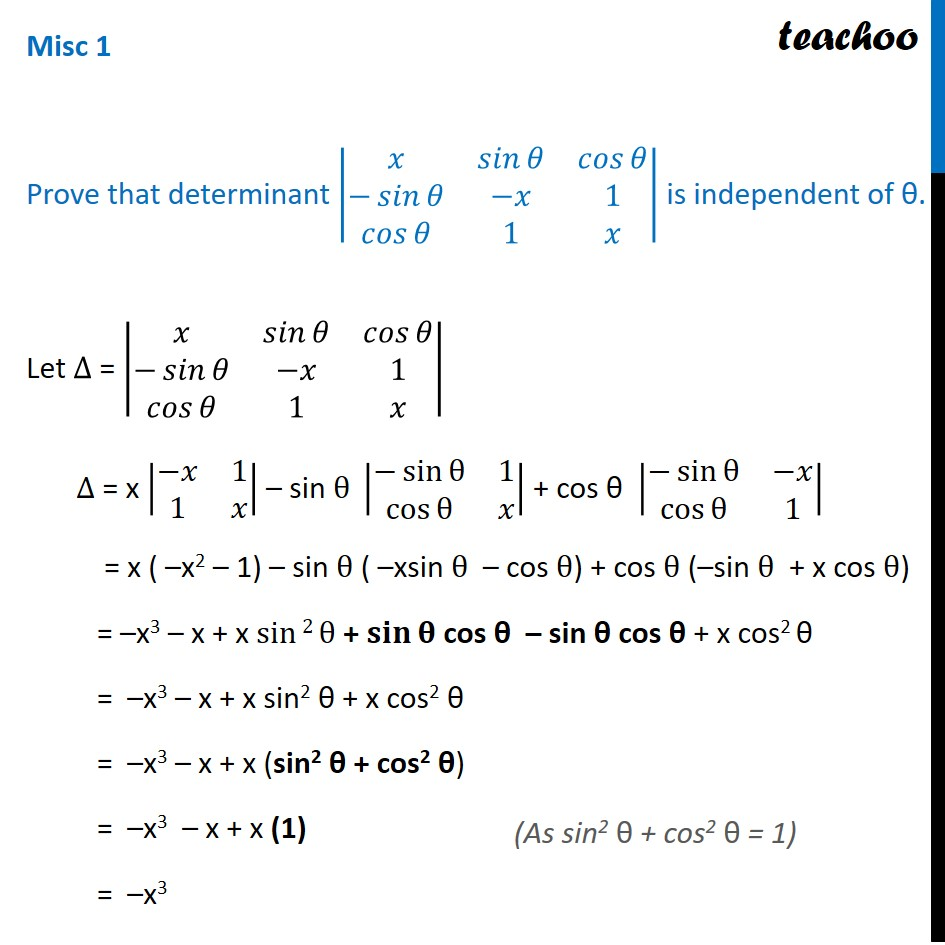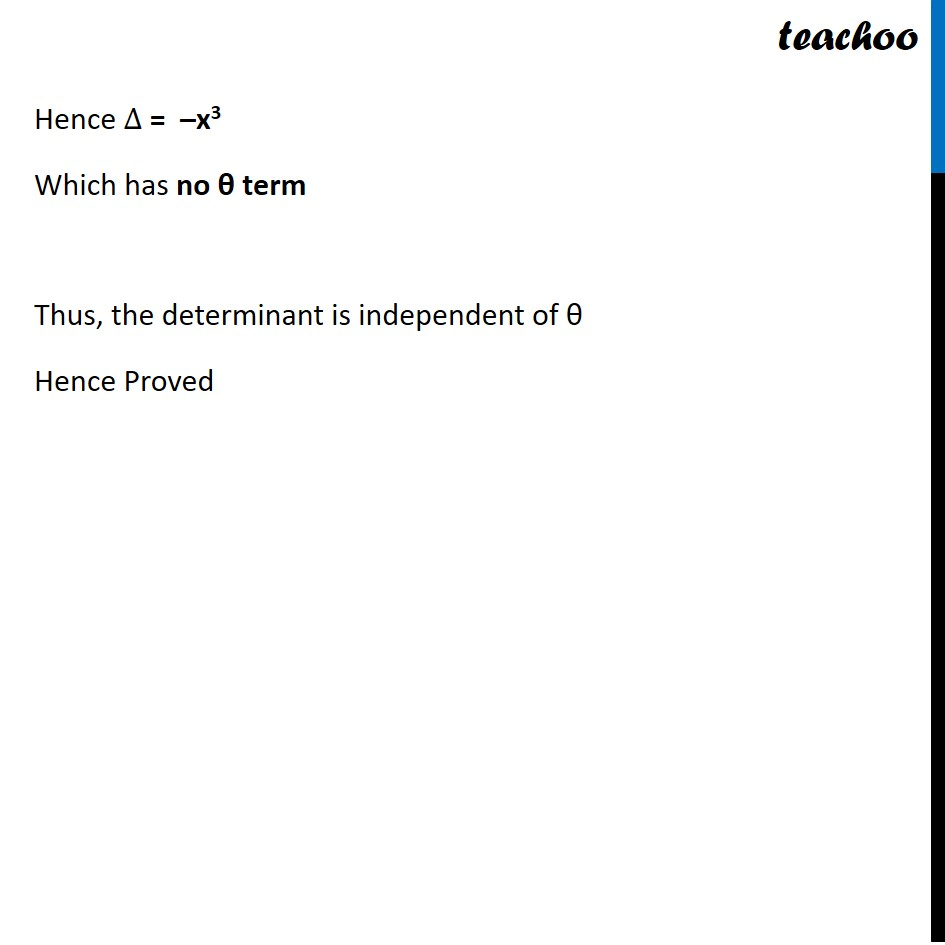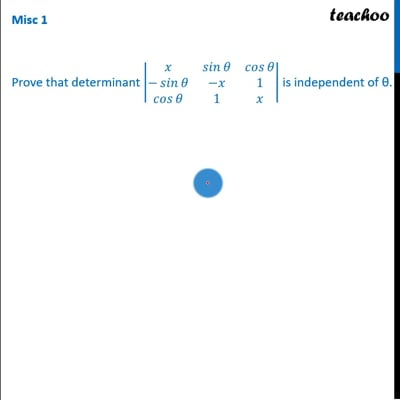Miscellaneous

Chapter 4 Class 12 Determinants
Serial order wiseThis video is only available for Teachoo black users

Solve all your doubts with Teachoo Black (new monthly pack available now!)

### Transcript

Misc 1 Prove that determinant |■8(𝑥&𝑠𝑖𝑛⁡𝜃&𝑐𝑜𝑠⁡𝜃@−𝑠𝑖𝑛⁡𝜃&−𝑥&1@𝑐𝑜𝑠⁡𝜃&1&𝑥)| is independent of θ. Let ∆ = |■8(𝑥&𝑠𝑖𝑛⁡𝜃&𝑐𝑜𝑠⁡𝜃@−𝑠𝑖𝑛⁡𝜃&−𝑥&1@𝑐𝑜𝑠⁡𝜃&1&𝑥)| ∆ = x |■8(−𝑥&1@1&𝑥)| – sin θ |■8(−sin⁡θ&1@cos⁡θ&𝑥)| + cos θ |■8(−sin⁡θ&−𝑥@cos⁡θ&1)| = x ( –x2 – 1) – sin θ ( –xsin θ – cos θ) + cos θ (–sin θ + x cos θ) = –x3 – x + x sin⁡〖2 θ〗 + 𝐬𝐢𝐧⁡𝛉 cos θ – sin θ cos θ + x cos2 θ = –x3 – x + x sin2 θ + x cos2 θ = –x3 – x + x (sin2 θ + cos2 θ) = –x3 – x + x (1) = –x3 (As sin2 θ + cos2 θ = 1) Hence ∆ = –x3 Which has no θ term Thus, the determinant is independent of θ Hence Proved